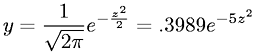Equations > Statistics > Distributions > Standard Normal Distribution

### Standard Normal DistributionLatex Code:

MathML Code:

 $y=\frac1\sqrt{2\pi }{e}^{-\frac{z}^{2}2}=.3989{e}^{-5{z}^{2}}$

MathType 5.0: